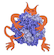IMP  2.3.1 The Integrative Modeling Platform
IMP.pmi.output Namespace Reference

Classes for writing output files and processing them. More...

## Detailed Description

Classes for writing output files and processing them.

## Classes

class  Output
Class for easy writing of PDBs, RMFs, and stat files. More...

class  ProcessOutput
A class for reading stat files. More...

## Functions

def plot_field_histogram
This function is plotting a list of histograms from a value list. More...

def plot_fields_box_plots
This function plots time series as boxplots fields is a list of time series, positions are the x-values valuename is the y-label, positionname is the x-label. More...

## Function Documentation

 def IMP.pmi.output.plot_field_histogram ( name, values_lists, valuename = None, bins = 40, colors = None, format = 'png', reference_xline = None, yplotrange = None, xplotrange = None, normalized = True, leg_names = None )

This function is plotting a list of histograms from a value list.

Parameters
 name the name of the plot value_lists the list of list of values eg: [[...],[...],[...]] valuename=None the y-label bins=40 the number of bins colors=None. If None, will use rainbow. Else will use specific list format="png" output format reference_xline=None plot a reference line parallel to the y-axis yplotrange=None the range for the y-axis normalized=True whether the histogram is normalized or not leg_names names for the legend

Definition at line 747 of file output.py.

 def IMP.pmi.output.plot_fields_box_plots ( name, values, positions, frequencies = None, valuename = 'None', positionname = 'None', xlabels = None )

This function plots time series as boxplots fields is a list of time series, positions are the x-values valuename is the y-label, positionname is the x-label.

Definition at line 812 of file output.py.